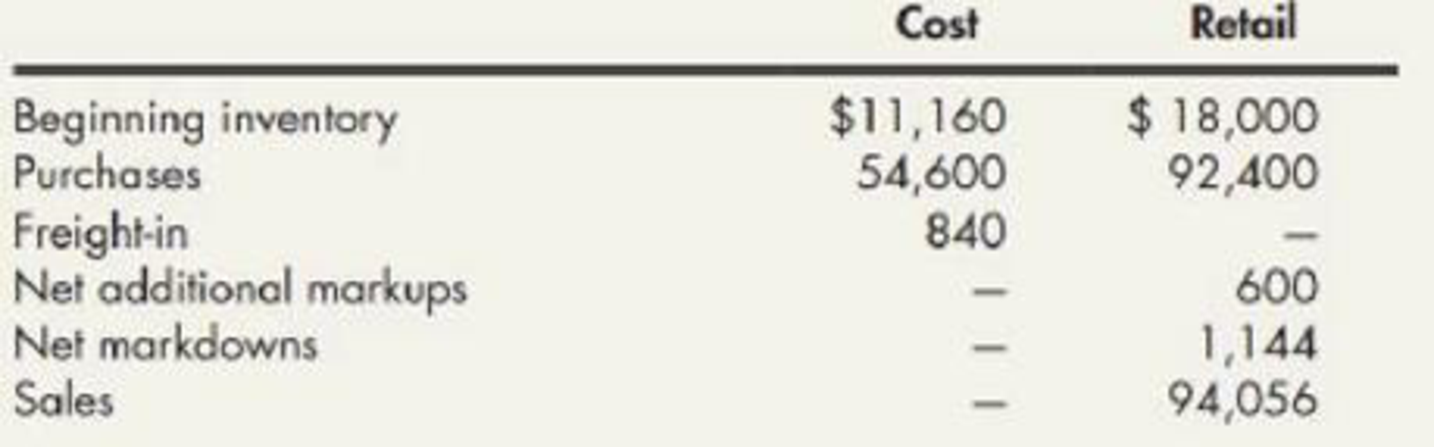Chapter 8, Problem 13E### Intermediate Accounting: Reporting...

3rd Edition
James M. Wahlen + 2 others
ISBN: 9781337788281

#### Solutions

Chapter
Section### Intermediate Accounting: Reporting...

3rd Edition
James M. Wahlen + 2 others
ISBN: 9781337788281
Textbook Problem
18 views

# Retail Inventory Method The following information relates to the retail inventory method used by Jeffress Company:Required: 1. Compute the ending inventory by the retail inventory method using the following cost flow' assumptions (round the cost-to-retail ratio to 3 decimal places): a. FIFO b. average cost c. LIFO d. lower of cost or market (based on average cost) 2. Next Level What assumptions are necessary for the retail inventory method to produce accurate estimates of ending inventory?

1.

To determine

Calculate the cost of ending inventory by the retail method using FIFO, average cost, LIFO, and LCM cost flow assumptions.

Explanation

Retail inventory method: It takes into account all the retail amounts that is, the current selling prices. Under this method, the goods available for sale, at retail is deducted from the sales, at retail to determine the ending inventory, at retail.

Conventional Retail Method: Conventional retail method refers to the estimation of the lower of average cost or market by eliminating the markdowns from the calculation of the cost-to-retail percentage.

In this case, the cost-to-retail percentage will be determined by dividing the goods available for sale at cost by the goods available for at retail (excluding markdowns). Thus, the conventional retail method will always result in lower estimation of ending inventory when the markdowns exist.

a.

FIFO: Under this inventory method, the units that are purchased first are sold first. Thus, it starts from the selling of the beginning inventory, followed by the units purchased in a chronological order of their purchases took place during a particular period.

Calculate the cost of ending inventory by the retail method using FIFO cost flow.

 Ending Inventory - FIFO Details Cost ($) Retail ($) Purchases 54,600 92,400 Freight-in 840 Markups (net) 600 Markdowns 0 (1,144) 55,440 91,856 Add: Beginning inventory 11,160 18,000 Goods available for sale 66,600 109,856 Less: Sales (94,056) Ending inventory at retail $15,800 Ending inventory at cost$9,543

Table (1)

Working note 1:

Calculate ending inventory at cost:

Step 1: Calculate cost-to-retail ratio.

Cost-to-retail ratio= (Goods available for sale at cost (After markdowns)Goods available for sale at retail (After markdowns))=($55,440$91,856)=.604

Step 2: Calculate ending inventory at cost.

Ending inventory at cost = (Ending inventory at retail×Cost-to-retail ratio)=($15,800×.604)=$9,543

b.

Average cost method: Under this method, the cost of the goods available for sale is divided by the number of units available for sale during a particular period.

Calculate the cost of ending inventory by the retail method using average cost flow.

 Ending Inventory - Average Cost Details Cost ($) Retail ($) Beginning inventory 11,160 18,000 Add:  Net purchase 54,600 92,400 Freight in 840 Net markups (net) 600 Less:  Net markdowns 0 (1,144) Goods available for sale 66,600 109,856 Less: Sales (94,056) Estimated ending inventory at retail $15,800 Estimated ending inventory at cost$9,575

Table (2)

Working note 1:

Calculate ending inventory at cost.

Step 1: Calculate cost-to-retail ratio.

Cost-to-retail ratio= (Goods available for sale at cost (After markdowns)Goods available for sale at retail (After markdowns))=($66,600$109,856)=.606

Step 2: Calculate ending inventory at cost.

Ending inventory at cost = (Ending inventory at retail×Cost-to-retail ratio)=($15,800×.606)=$9,574

c

2.

To determine

Indicate the needed assumptions for the retail inventory method to produce correct estimated of ending inventory.

### Still sussing out bartleby?

Check out a sample textbook solution.

See a sample solution

#### The Solution to Your Study Problems

Bartleby provides explanations to thousands of textbook problems written by our experts, many with advanced degrees!

Get Started